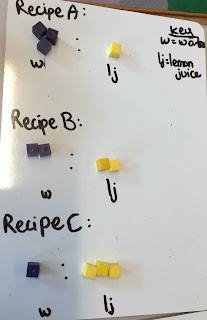## Monday, 14 March 2016

### Visualising Ratios

Today, we looked at how visualising and creating tables can be a useful strategy when using ratios for problem solving.

Children need opportunities to visualise maths and since the concept of ratios can be a bit tricky for some, I gathered a group together and we explored visualising ratios.

We began by thinking of making lemonade:Photo Source: http://www.simplyrecipes.com/recipes/perfect_lemonade/

We brainstormed the following ingredients we needed:

°   water
°   squeezed lemon juice
°   sugar

We then came up with three possible recipes:

RECIPE A:      You put in 3 parts water to 1 part lemon juice.

RECIPE B:       You put in 2 parts water to 2 parts lemon juice

RECIPE C:       You put in 1 part water to 3 parts lemon juice.

What do these ratio recipes look like?

Children created ways to visualise them using cubes. After creating our display we looked at other approaches and discussed the different styles:

We thought this approach made seeing            We liked the idea of including
the relationship between the ratio                    a key so the viewer would
values very easy:                                           have a clearer understanding:We liked how this student                  We thought the detail made it clear
showed the ratios written in               to understand, but having the cubes so
different ways:                                  far apart didn't help us see the ratio
relationships well:Looking at our ratio cubes, we felt it was easy to see which lemonade recipe would be the strongest and the weakest.

To help visualise what we do when we find equivalent ratio proportions, we then used the cubes to show what our lemonade would look like if we doubled, halved or tripled the portion amounts:Again we looked at the different approaches and discussed the strengths in each.

I then introduced the strategy of using a table to help visualise and solve ratio word problems.

Eg,  When making recipe A, if we had 12 parts lemon juice, how many parts water would be needed for the same ratio.

We then explored how a table can be a useful strategy when solving ratio word problems. We imagined we had different parts of either lemon juice or water and asked our table partners how much would be needed at the same ratio.

We shared and discussed the different strategies we used and wondered if some of them could be used with all types of similar ratio situations or not.

One student wondered what we could do if we knew how much one part equalled.  It was a great question so we tried it out.

Some of us imagined 1 part = 50 mL, others imagined 1 part = 0.75mL etc

Using their measurement, they then created a visual column graph and a table to help solve how much of each would be needed given a different ratio amount.

In our class reflection, we felt creating tables helps us to see the relationships between the two values and also it makes it easier to solve them. We also wondered if there were other useful strategies like a table to help us with ratios so we added that to our wonder wall to find out as our unit progresses.

This guided enquiry is a useful teaching strategy especially for students new to a concept. It gives them tools they can use for later in our unit when they start creating their own enquiries into ratios.